Latest Banking jobs   »   Reasoning Ability Quiz For SBI Clerk...

# Reasoning Ability Quiz For SBI Clerk Prelims 2022- 29th May

Directions (1-5): Study the following arrangement carefully and answer the questions given below.
Some people are sitting in two parallel rows facing each other. A, B, C, D, E, F and G are sitting in row 1 facing north and P, Q, R, S, T, U and V are sitting in row 2 facing south (not necessarily in the same order).
Three persons sit between B and C and one of them sits at an end. Q sits fourth to the right of T. P sits to the immediate left of U, who sits at the middle of the row. A is not a neighbor of B. E sits to the immediate right of the one who faces P. D faces the one who sits second to the left of V. S faces G. R faces the one who sits to the immediate right of A. T does not sit at any end.

Reasoning Ability Quiz For SBI Clerk Prelims 2022 CHECK IN HINDI

Q1. Who among the following sits second to the left of the one who is facing T?
(a) F
(b) E
(c) C
(d) A
(e) None of these

Q2. How many persons sit between C and the one who faces P?
(a) None
(b) One
(c) Two
(d) Three
(e) More than three

Q3. Who among the following faces the person who sits diagonally opposite to S?
(a) G
(b) C
(c) V
(d) Q
(e) T

Q4. Who among the following pair sits at the extreme end of the row?
(a) G, B
(b) B, S
(c) V, C
(d) S, E
(e) None of these

Q5. What is the position of P with respect to Q?
(a) Third to the left
(b) Third to the right
(c) Second to the left
(d) Immediate right
(e) Immediate left

Directions (6-10): Study the following information to answer the given questions:
In a certain code language

‘ancient scientist sun’ is written as ‘la ac df’,
‘ancient moon heat crystal pink’ is written as ‘oq pr rs ac tp’,
‘new scientist moon heat queen’ is written as ‘tp df rs ge hg’
‘space moon pink’ is written as ‘rt tp pr’.

Q6. What is the code for ‘pink’?
(a) oq
(b) rs
(c) pr
(d) ac
(e) tp

Q7. ‘space heat moon crystal’ could be coded as?
(a) tp rt rs oq
(b) rt ac rs ge
(c) df rt rs oq
(d) rt ac pr oq
(e) None of these

Q8. Which of the following word represent ‘hg’ in coding language?
(a) scientist
(b) moon
(c) queen
(d) new
(e) Either ‘new’ or ‘queen’

Q9. Which of the following may be the code for ‘keep moon sun’?
(a) tp la rt
(b) la df oq
(c) lb la tp
(d) ge la ac
(e) None of these

Q10. ‘tp oq pr’ is the code for?
(a) heat moon pink
(b) space moon pink
(c) moon crystal pink
(d) Can’t be determined
(e) None of these

Directions (11-15): Study the following arrangement carefully and answer the questions given below.

G 4 N E 3 L M % J K @ H I © A 5 T 1 W \$ X 2 Y S 6 # F 9 D R 8 € U Z C & O

Q11. How many such numbers are there in the given arrangement, each of which is immediately preceded by a vowel and not immediately followed by a symbol?
(a) None
(b) One
(c) Two
(d) Three
(e) More than three

Q12. Which of the following element is the 5th to the left of the 12th from the right end of the given arrangement?
(a) 2
(b) #
(c) X
(d) \$
(e) Y

Q13. How many such symbols are there in the given arrangement, each of which is immediately followed by a letter but not immediately preceded by a number?
(a) None
(b) One
(c) Two
(d) Three
(e) More than three

Q14. Which of the following element is 7th to left of 17th from left end?
(a) 2
(b) K
(c) X
(d) \$
(e) Y

Q15.How many vowels are there in above arrangements which is immediately followed by vowel?
(a) None
(b) One
(c) Two
(d) Three
(e) None of these

Solutions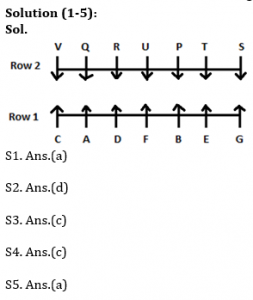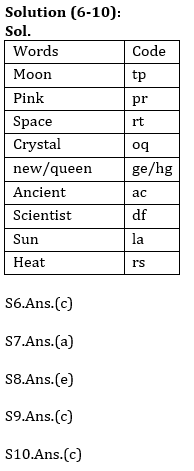S11. Ans.(c)
Sol. E3L, A5T

S12. Ans.(c)
Sol. 5th to the left of the 12th from the right end= 17th from the right end= X

S13. Ans.(e)
Sol. M%J, K@H, I©A, W\$X, C&O

S14. Ans.(b)
Sol. 7th to left of 17th from left end= 10th from the left end= K

S15. Ans.(a)#### Congratulations!Incorrect details? Fill the form again here

•Reasoning Ability Quiz For IBPS RRB PO P...
•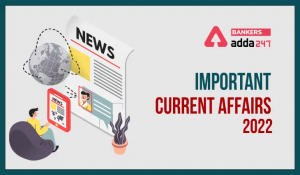Important Current Affairs Quiz for Bank ...
•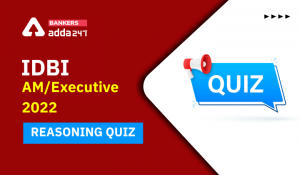Reasoning Ability Quiz For IDBI AM/Execu...
•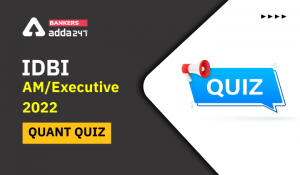Quantitative Aptitude Quiz For IDBI AM/E...
•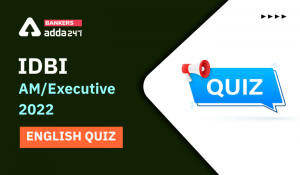English Quizzes For IDBI AM/Executive 20...
•Reasoning Ability Quiz For IBPS RRB PO P...